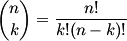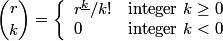# Binomial coefficients

The simplest definition of binomial coefficients is given bywhere n is a positive integer and 0 ≤ kn. There are two generalizations of this definition. The first is to let n be any real number. Then the binomial coefficient (r, k) can be defined aswhereis the kth falling power of r. This definition is adequate for most applications.

The final generalization is to let z and w be any complex numbers and define the binomial coefficient (z, w) asThis generalization is more complicated, but in some sense it is more natural. Essentially it uses the simplest definition and replaces n! with Γ(n+1). However, the business of the limits is subtle and important.

For motivation of these definitions and details regarding how they work, see the article Binomial coefficients.